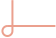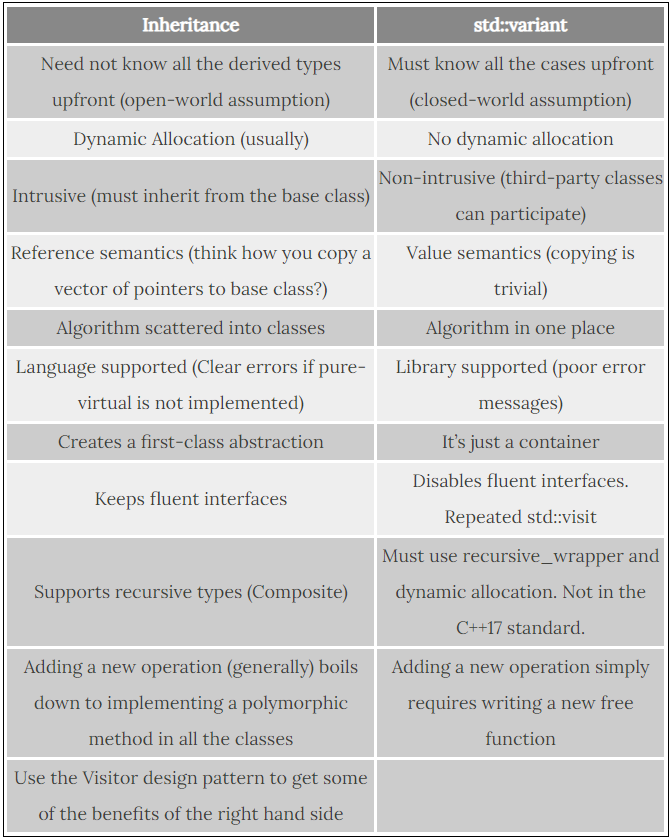• 发文
• 评论
• 微博
• 空间
• 微信

# 高端操作:不用继承我也能实现多态variant是什么？

为什么要引入variant？

如何确定variant中当前存放的数据类型？

variant为什么要搭配monostate？

如何用variant实现多态？

variant这货类似于union，可以存放多种类型的数据，但任何时刻最多只能存放其中一种类型。

union MyUnion {    int a;    float b;    double c;};
void test_simple_union() {    MyUnion u;    u.a = 1;    std::cout << u.a="">"\n"

;
u.b = 1.32f;    std::cout << u.b="">"\n"

;
u.c = 2.32;    std::cout << u.c="">"\n"

;}

union貌似也只能这么使用，没有其他方法。

• struct A {    A() = default;    A(int aa) : a{aa} { std::cout <<>"A() \n"

; }    ~A() { std::cout <<>"~A() \n"

; }    int a;};
struct B {    B() = default;    B(float bb) : b{bb} { std::cout <<>"B() \n"

; }    ~B() { std::cout <<>"~B() \n"

; }    float b;};
union MyStructUnion {    A a;    B b;    /**     * @brief 在析构函数中我要做什么？不知道当前类型究竟是A还是B     * 那调用 a.~A() 还是 b.~B() ?     */    ~MyStructUnion() { std::cout <<>"~MyStructUnion() \n"

; }};
/** * @brief 需要手动调用析构函数 * */void test_struct_union() {    MyStructUnion u;
new (&u.a) A(1);    std::cout << u.a.a="">"\n"

;    u.a.~A();
u.b = B(2.3f);    std::cout << u.b.b="">"\n"

;    u.b.~B();}

; }    ~C() { std::cout <<>"~C() \n"

; }    std::string c;};
/** * @brief 使用variant完全不需要手动调用构造和析构函数，它会自动处理好所有逻辑，非常方便 * */void test_variant() {    std::variant u; ///< 下面很快就会介绍monostate    u = 1;    std::cout <<>std::get(u).a <<>"\n";    u = std::string("dsd");    std::cout <<>std::get(u).c <<>"\n";}

void test_variant() {    std::variant var;    var = 12;    std::cout <<>std::get(var) <<>"\n";    var = 12.1f;    std::cout <<>std::get(var) <<>"\n";}

void test_monostate2() {    ///< 编译失败，S如果没有构造函数，需要加monostate    std::variant var;    var = 12;    std::cout <<>std::get(var).value <<>"\n";}

void test_monostate() {    std::variant var;    var = 12;    std::cout <<>std::get(var).value <<>"\n";}

template struct get_index_impl {};
template struct get_index_impl :,> std::integral_constant {};
template struct get_index_impl :,> get_index_impl1, T, Ts...> {};
template struct get_index> :,>get_index_impl<0, T, Ts...> {};
template constexpr auto get_index_v = get_index::value;,>
using variant_t = std::variant;
constexpr static auto kPlaceholderIndex = get_index_v;constexpr static auto kIntIndex = get_index_v;constexpr static auto kFloatIndex = get_index_v;constexpr static auto kStringIndex = get_index_v;

auto custom_visitor = [](const auto& value) {        switch (value.index()) {            case kPlaceholderIndex:                std::cout <<>"placehodler value "                          <<>"\n";                break;            case kIntIndex:                std::cout <<>"int value " <<>std::get(value) <<>"\n";                break;            case kFloatIndex:                std::cout <<>"float value " <<>std::get(value) <<>"\n";                break;            case kStringIndex:                std::cout <<>"string value " <<>std::get(value) <<>"\n";                break;        }    };    variant_t var;    custom_visitor(var);
var = 1;    custom_visitor(var);
var = 2.90f;    custom_visitor(var);
var = std::string("hello world");    custom_visitor(var);
var = std::string("hello type");}
int main() { test_using_index(); }

void operator()(float f) const { std::cout <<>"float " << f="">"\n"; }
void operator()(std::string s) const { std::cout <<>"string " << s="">"\n"; }};
void test_visitor_functor() {    std::variant var;    var = 1;    std::visit(Visitor(), var);    var = 2.90f;    std::visit(Visitor(), var);    var = std::string("hello world");    std::visit(Visitor(), var);}
// 输出int 1float 2.9string hello world

visit内部会自动判断当前variant内部存储的类型，进而触发不同的行为。

// 输出value 1value 2.9value hello worldstring value hello type

struct B {    void func() const { std::cout <<>"func B \n"; }};
struct CallFunc {    void operator()(const A& a) { a.func(); }    void operator()(const B& b) { b.func(); }};
void test_no_param_polymorphism() {    std::variant,>    var = A();    std::visit(CallFunc{}, var);    var = B();    std::visit(CallFunc{}, var);}

struct D {    void func(int value) const { std::cout <<>"func D " << value="">"\n"; }};
struct CallFuncParam {    void operator()(const C& c) { c.func(value); }    void operator()(const D& d) { d.func(value); }
int value;};
void test_param_polymorphism() {    std::variantvar;,>   var = C();    std::visit(CallFuncParam{1}, var);    var = D();    std::visit(CallFuncParam{2}, var);}https://www.cppstories.com/2020/04/variant-virtual-polymorphism.html/

https://www.cppstories.com/2018/06/variant/

http://cpptruths.blogspot.com/2018/02/inheritance-vs-stdvariant-based.html

https://github.com/chengxumiaodaren/cpp-learning/tree/master/src/varian

0